4530.0 - Crime Victimisation, Australia, 2014-15
ARCHIVED ISSUE Released at 11:30 AM (CANBERRA TIME) 17/02/2016
 Page tools: .mffeedback,#pjs { display :none; } Enable Javascript to Print PagesPrint All Explanatory Notes Glossary Abbreviations Quality Declaration Data Quality (Technical Note) TECHNICAL NOTE RELIABILITY OF THE ESTIMATES 1 The estimates in this publication are based on information obtained from a sample survey. Errors in data collection or processing, known as non-sampling error, can impact on the reliability of the resulting statistics. In addition, estimates based on sample surveys are subject to sampling error. That is, the estimates may differ from the true value of the characteristics being measured that would have been obtained had all persons in the population been included in the survey. Non-sampling error 2 Non-sampling error may occur in any statistical collection, whether it is based on a sample or a full count such as a census. Sources of non-sampling error include non-response, errors in reporting by respondents or recording of answers by interviewers and errors in coding and processing data. Every effort is made to reduce non-sampling error by careful design and testing of questionnaires, training and supervision of interviewers, and extensive editing and quality control procedures at all stages of data processing. Sampling error 3 Sampling error refers to the difference between an estimate obtained from surveying a sample of persons, and the true value of the characteristic being measured that would have been obtained if the entire in-scope population was surveyed. Sampling error can be measured in a standardised way using Standard Error (SE) calculations, which indicates the extent to which an estimate might have varied by chance because only a sample of persons was surveyed. There are about two chances in three (67%) that a sample estimate will differ by less than one SE from the number that would have been obtained if all persons had been surveyed, and about 19 chances in 20 (95%) that the difference will be less than two SEs. 4 In this publication, the standard error of the estimate is given as a percentage of the estimate it relates to, known as the relative standard error (RSE).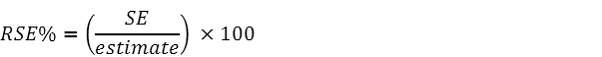5 RSEs for all estimates have been calculated using the Jackknife method of variance estimation. This involves the calculation of 30 'replicate' estimates based on 30 different subsamples of the obtained sample. The variability of estimates obtained from these subsamples is used to estimate the sample variability surrounding the estimate. 6 The Excel files available from the Downloads tab contain all the tables produced for this release, including all estimates and their corresponding RSEs. 7 Only estimates (numbers or percentages) with RSEs less than 25% are considered sufficiently reliable for most analytical purposes. However, estimates with RSEs over 25% have also been included. Estimates with an RSE in the range 25% to 50% are less reliable and should be used with caution, while estimates with RSEs greater than 50% are considered too unreliable for general use. All cells in the publication tables containing an estimate with an RSE of 25% or over have a cell comment attached, indicating whether the RSE of the estimate is in the range 25-49% or is over 50%. These cells can be identified by a red indicator in the corner of the cell. The comment appears when the mouse pointer hovers over the cell. Calculation of Standard Error 8 Standard error (SE) can be calculated using the estimate (count or percentage) and the corresponding RSE. For example, Table 1 shows that the estimated number of persons who experienced physical assault in the last 12 months was 400,400 with a corresponding RSE of 4.9%. The SE (rounded to the nearest 100) is calculated by: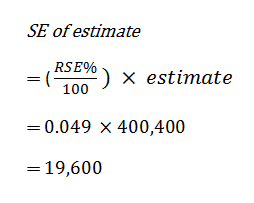9 Therefore, there is about a two in three chance that the true value, which would have been obtained had all persons been included in the survey, falls within the range of one standard error below to one standard error above the estimate (380,800 to 420,000), and about a 19 in 20 chance that the true value falls within the range of two standard errors below to two standard errors above the estimate (361,200 to 439,600). This example is illustrated in the diagram below: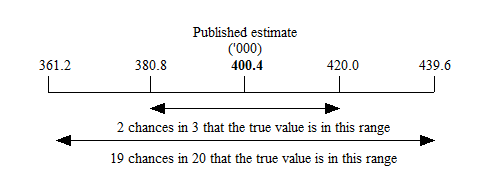Proportions and Percentages 10 Proportions and percentages formed from the ratio of two estimates are also subject to sampling errors. The size of the error depends on the accuracy of both the numerator and the denominator. A formula to approximate the RSE of a proportion is given below. This formula is only valid when x is a subset of y: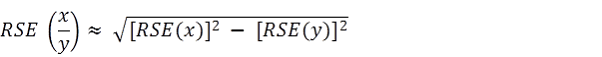11 As an example, using data from Table 8, 192,600 persons experienced a single incident of physical assault, representing 48.1% of all persons who experienced physical assault. The RSE for the number of persons experiencing one incident of physical assault is 6.4% and the RSE for the total number of persons experiencing physical assault is 4.9%. Applying the above formula, the RSE of the proportion is: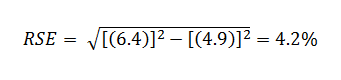12 Using the formula given in technical note 8 above, the standard error (SE) for the proportion of persons experiencing physical assault who experienced a single incident is 2.0% (0.042 x 48.1). Hence, there are about two chances in three that the true proportion of persons experiencing physical assault who experienced a single incident is between 46.1% and 50.1%, and 19 chances in 20 that the true proportion is between 44.1% and 52.1%. Differences 13 Standard error can also be calculated on the difference between two survey estimates (counts or percentages). The standard error of the difference between two estimates is determined by the individual standard errors of the two estimates and the relationship (correlation) between them. An approximate standard error of the difference between two estimates (x,y) can be calculated using the following formula: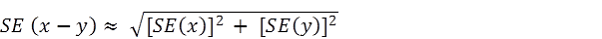14 While this formula will only be exact for differences between separate and uncorrelated characteristics or sub populations, it provides a good approximation for the differences likely to be of interest in this publication. Significance Testing 15 The difference between two survey estimates can be tested for statistical significance, in order to determine the likelihood of there being a real difference between the populations with respect to the characteristic being measured. The standard error of the difference between two survey estimates (x and y) can be calculated using the formula shown above in technical note 13. This standard error is then used in the following formula to calculate the test statistic: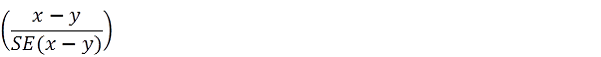16 If the value of the test statistic is greater than 1.96 then this supports, with a 95% level of confidence, a real difference (i.e. statistically significant) between the two populations with respect to the characteristic being measured. If the test statistic is not greater than 1.96, it cannot be stated with confidence that there is a real difference between the populations with respect to that characteristic. 17 Annual changes between 2013-14 and 2014-15 in victimisation rates, reporting rates, and the proportion of persons believing alcohol or any other substance contributed to their most recent incident of physical assault and face-to-face threatened assault, have been tested to determine whether the change is statistically significant. Significant differences have been annotated with a cell comment. In all other tables which do not show the results of significance testing, users should take account of RSEs when comparing estimates for different populations, or undertake significance testing using the formula provided in technical note 15 to determine whether there is a statistical difference between any two estimates. Changes in Crime Victimisation Rates Over Time 18 For the first time in 2014-15, changes in crime victimisation rates over time were examined by fitting functions to the seven time points of data for each crime type. The function of best fit was selected based on the highest R-squared value. R-squared is a measure of how well the fitted model explains the variance of the dependant variable (i.e. the crime type). The closer R-squared is to 1.0, the better the function fits the data points. 19 For the modelled data presented in this publication, only fitted functions with a R-squared value greater than 0.8 have been included. Whilst the R-squared has limitations, particularly in the context of non-linear models, it still is a useful indicator of model fit. 20 The functions used to analyse patterns of change in national victimisation rates over time have not taken into account the survey error associated with the annual victimisation rates. This is expected to have a negligible impact on the analysis, as the survey error is small. 21 The functions used to analyse patterns of change in jurisdictional victimisation rates over time have not taken into account the survey error associated with the annual victimisation rates and fitted functions have only been included where the following criteria were met: Survey estimates for the victimisation rate had a relative standard error (RSE) of 15% or less. The fitted function was within the 95% confidence interval for the victimisation rate and had an R-squared value of 0.8. Document Selection These documents will be presented in a new window.